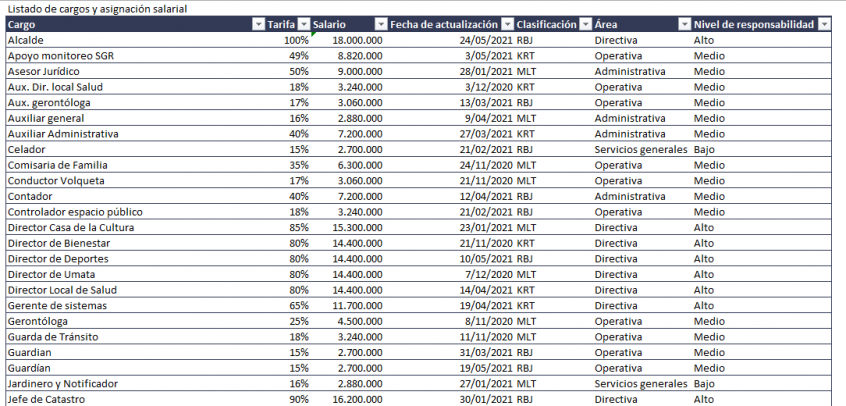Excel 2016: Vlookup – Return all entries in a column using vLookup joined by comma (NO TextJoin)Tabla de contenido

Tecnología:

Windows – Excel 2016

Context:

This is our data ⬇️

Data is formated as an structured Table (Excel Table)

Problem / Need:

Write in one cell all the matches in the “Cargo” column where “Área” is “Servicios generales”

Solution:

Formula → =CONCAT(IF(Tabla[Área]="Servicios generales",Tabla[Cargo]&",",""))

Explanation:

Tabla[Área]="Servicios generales"

This part of the formula evaluates each cell in the Area column and returns true for the ones that match the text

Tabla[Cargo]&","

This part of the formula returns the Cargo in the row of each of the matched cells and adds the comma at the end of each one

Note: The comma at the end is the key to replace the TextJoin formula that is not available until Excel version 2019 onwards

CONCAT(

The Concat function takes each result and writes them in the cell